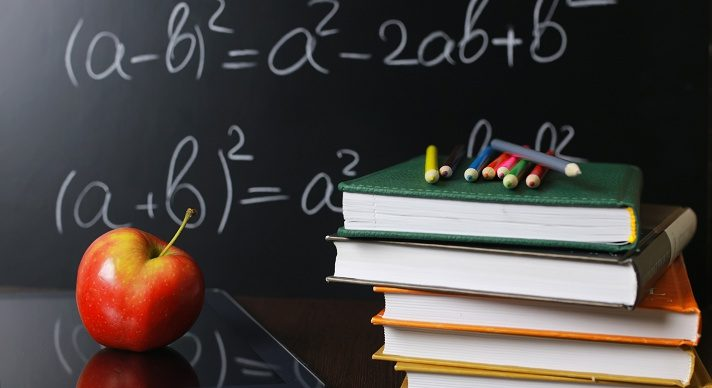# Algebra II-SE### Course Overview

The Acellus Algebra II course for Special Ed students focuses on the concepts most important for these students, digging in deeper than the regular Algebra II course to provide needed additional instruction for success. This is taught by award-winning Acellus Master Teacher, Patrick Mara.This course was developed by the International Academy of Science. Learn More

### Scope and Sequence

Unit 1 In this unit, students learn about algebraic expressions, explore sets of numbers, learn about simplifying and evaluating, and investigate solving equations and inequalities. They also study absolute value. Unit 2 In this unit students come to understand the definition of a function, they learn about direct variation, they study slope-intercept, they investigate point-slope, and they learn about piece-wise defined functions. In addition, they explore transformations of functions, learn about absolute value functions, and learn to work with inequalities in two variables. Unit 3 In this unit students explore solving graphically.  They also learn about substitution and elimination, and study systems of inequalities. Unit 4 In this unit students learn about transformations, come to understand standard form and factoring, work with quadratic equations, and explore completing the square. They also study the quadratic formula and complex numbers. Unit 5 In this unit students learn about polynomial functions. They study factored form, explore polynomial equations, gain understanding of dividing polynomials, and investigate synthetic division. Unit 6 In this unit students gain understanding of properties of exponents. They also study exponential functions. Unit 7 In this unit students study probability. They also learn about analyzing data, as well as samples and surveys. Unit 8 In this unit, students learn the definition of periodic. They also come to understand the unit circle. They study radian measure, and explore the sine, cosine, and tangent functions. They learn to work with reciprocal trigonometric functions and basic identities, as well as with inverse trig functions and right triangles.